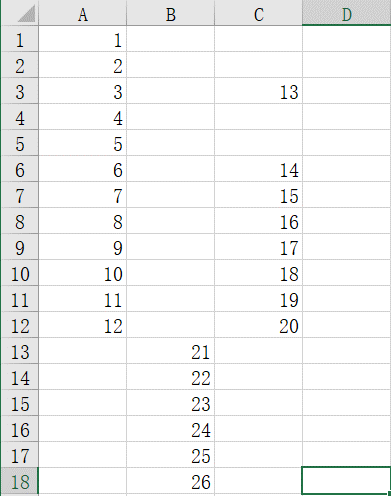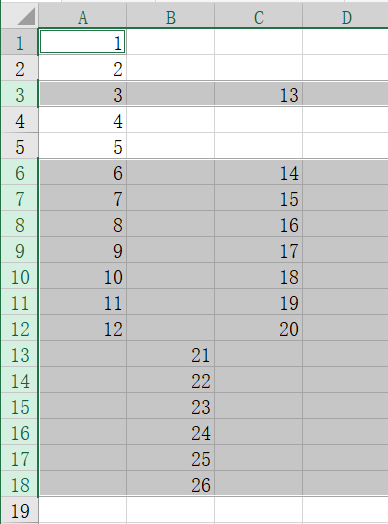• 定位单元格 一、使用Excel定位对话框 在Excel自带功能中，即带有根据特殊格式定位的功能：【开始】→【查找与选择】→【定位条件】。可以根据需要定位查找“标注”、“常量”、“公式”、“空值”、“可见单元格”等...
• 1.EXCEL VBA遍布文件夹的操作 关于VBA遍历文件夹主要用的是提供的Application.FileDialo函数来由个人进行自由选择，通过获取选择的文件夹地址之后，通过Dir函数来匹配选取文件夹下的相应的文档。相应的VBA程序代码...

1.EXCEL VBA遍布文件夹的操作

关于VBA遍历文件夹主要用的是提供的Application.FileDialo函数来由个人进行自由选择，通过获取选择的文件夹地址之后，通过Dir函数来匹配选取文件夹下的相应的文档。相应的VBA程序代码如下：

Dim sel_Path As String   '//定义一个选择的文件夹
Dim MyFile As String    '//文件夹中符合条件的文件

'//选取相应的文件夹
With Application.FileDialog(msoFileDialogFolderPicker)

.Title = "请选择文件夹"

If .Show = -1 Then
sel_Path = .SelectedItems(1)       '//所选择的文件夹路径
Else
MsgBox "已取消操作！"
Exit Sub
End If

End With

Dim sel_PathFullName As String                            '定义一个文件的全路径名称

MyFile = Dir(sel_Path & "\" & "*.csv")

2.关于单元格地址的获取

在写VBA的过程中，对于单元格地址的获取至关重要，它直接决定了能否完成正确的完成相应的操作。获取单元格地址主要有以下几种方法：

2.1          以下几种获取结果如注释所示

F_Max_Range = ActiveCell.Address()           '获得单元格的地址，形式为$A$1
F_Max_Range = ActiveCell.Address(0, 1)       '获得单元格的地址，形式为$A1 F_Max_Range = ActiveCell.Address(1, 0) '获得单元格的地址，形式为A$1
F_Max_Range = ActiveCell.Address(1, 1)       '获得单元格的地址，形式为$A$1

2.2        以变量的形式获取单元格地址

以获取最后一列的最后一个单元格的地址为例：首先需要获取获取最后一列的地址，再得到总的行数，将以上两种结果进行拼接，即得出最后一列的最后一个单元格的地址。相应的关键代码如下：

Num_Col = Wb.Worksheets(2).UsedRange.Columns.count          '总的列数
Row_Col = Wb.Worksheets(2).UsedRange.Rows.count             '总的行数

Add_Max_Col = Split(Cells(1, Num_Col).Address, "$")(1) '获得最后一列的地址 Range_Add_Max_Col = Add_Max_Col & "1" '最后一列的第一个单元格的地址 Range_Add_Max_Row = Add_Max_Col & Row_Col '最后一列的最后一个单元格的地址 2.3 关于单元格的偏移 单元格偏移主要用到的函数是Offset，如偏移1个和2个单元格的关键代码： F_Max_Range = ActiveCell.Offset(0, 1).Address(0, 0) S_Max_Range = ActiveCell.Offset(0, 2).Address(0, 0) 2.4 关于选择多个单元格 选择多个单元格要注意单元格之间的拼接格式，要注意中间的“：”，如对以上F_Max_Range 到 S_Max_Range单元格的选择的关键代码: Wb.Worksheets(2).Range(F_Max_Range & ":" & S_Max_Range).Select 3.关于复制和粘贴 复制和粘贴极大的简化了我们的工作，VBA的复制主要通过copy函数来实现，粘贴主要通过Paste来实现。要注意复制和粘贴之前 一定要选中所要复制的列。即select。关键代码： '提取出相应的列到sheet2表中 Dim sel_col As String '定义需要操作的列 sel_col = Workbooks("遍历文件夹中的csv文件（处理带逗号的VBA程序）").Worksheets(1).Range("B2").Value sel_col = Mid(sel_col, InStr(sel_col, ":") + 1) Wb.Worksheets(1).Range(sel_col).Select Selection.Copy Wb.Sheets.Add After:=ActiveSheet Wb.Worksheets(2).Select ActiveSheet.Paste 4 完全代码: 本完全代码实现了遍历相应文件夹下的所有EXCEL(CSV)文件，并复制相应的列到另一个sheet中。另外还有分列、绘图操作。关于EXCEL原文件暂不上传，读者可以根据需要进行相应的简单的修改即可。 '//遍历文件夹部分，并选中相应的csv文件 Dim sel_Path As String '//定义一个选择的文件夹 Dim MyFile As String '//文件夹中符合条件的文件 Dim count As Integer '//一共操作文件的数目 '//选取相应的文件夹 With Application.FileDialog(msoFileDialogFolderPicker) .Title = "请选择文件夹" If .Show = -1 Then sel_Path = .SelectedItems(1) '//所选择的文件夹路径 Else MsgBox "已取消操作！" Exit Sub End If End With '//创建一个新的文件夹用来保存数据处理的结果 On Error Resume Next Dim Save_Path_Name As String Save_Path_Name = sel_Path & "\" & "文件处理结果" VBA.MkDir (sel_Path & "\" & "文件处理结果") Dim sel_PathFullName As String '定义一个文件的全路径名称 Dim Wb As Workbook '定义一个要操作的工作薄 MyFile = Dir(sel_Path & "\" & "*.csv") '读入文件夹中的第一个.csv文件 Do While MyFile <> "" count = count + 1 '记录文件的个数 sel_PathFullName = sel_Path & "\" & MyFile '相应文件夹下的符合条件的csv文件 'sel_PathFullName = Application.GetOpenFilename '自定义文件的路径 Set Wb = Workbooks.Open(sel_PathFullName) '打开所选择的文件 'ActiveWindow.Visible = False 静默打开并不能读取文件 '提取出相应的列到sheet2表中 Dim sel_col As String '定义需要操作的列 sel_col = Workbooks("遍历文件夹中的csv文件（处理带逗号的VBA程序）").Worksheets(1).Range("B2").Value sel_col = Mid(sel_col, InStr(sel_col, ":") + 1) Wb.Worksheets(1).Range(sel_col).Select Selection.Copy Wb.Sheets.Add After:=ActiveSheet Wb.Worksheets(2).Select ActiveSheet.Paste Dim F_Numcol As Long '第一次复制后数据的列的数目 Dim F_Add_Max_Col As String '第一次复制后最后一列的地址 F_Numcol = Wb.Worksheets(2).UsedRange.Columns.count '选中最后一列 Wb.Worksheets(2).Columns(F_Numcol).Select F_Add_Max_Col = Split(Cells(1, F_Numcol).Address, "$")(1) & "1"       '获得最后一列的地址

'对列进行分列处理
TextQualifier:=xlDoubleQuote, ConsecutiveDelimiter:=False, Tab:=True, _
Semicolon:=False, Comma:=True, Space:=False, Other:=True, OtherChar:= _
"[", TrailingMinusNumbers:=True

Dim Num_Col As Long               '找出表中的最后一列
Dim Row_Col As Long               '找出表中的最后一行

Num_Col = Wb.Worksheets(2).UsedRange.Columns.count          '总的列数
Row_Col = Wb.Worksheets(2).UsedRange.Rows.count             '总的行数

Add_Max_Col = Split(Cells(1, Num_Col).Address, "$")(1) '获得最后一列的地址 Range_Add_Max_Col = Add_Max_Col & "1" '最后一列的第一个单元格的地址 Range_Add_Max_Row = Add_Max_Col & Row_Col '最后一列的最后一个单元格的地址 '选中最后一列 Wb.Worksheets(2).Columns(Num_Col).Select '对分列出的最后一列进行分列，从而删除"]"字符 Selection.TextToColumns Destination:=Range(Range_Add_Max_Col), DataType:=xlDelimited, _ TextQualifier:=xlDoubleQuote, ConsecutiveDelimiter:=False, Tab:=True, _ Semicolon:=False, Comma:=False, Space:=False, Other:=True, OtherChar _ :="]", TrailingMinusNumbers:=True '获得最后一个单元格右边的一个单元格并给其赋值V1 Wb.Worksheets(2).Range(F_Add_Max_Col).Select '最后一个单元格右边的一个单元格的地址 Dim F_Max_Range As String Dim S_Max_Range As String ' F_Max_Range = ActiveCell.Address() '获得单元格的地址，形式为$A$1 ' F_Max_Range = ActiveCell.Address(0, 0) '获得单元格的地址，形式为A1 ' F_Max_Range = ActiveCell.Address(0, 1) '获得单元格的地址，形式为$A1
'   F_Max_Range = ActiveCell.Address(1, 0)       '获得单元格的地址，形式为A$1 ' F_Max_Range = ActiveCell.Address(1, 1) '获得单元格的地址，形式为$A$1 F_Max_Range = ActiveCell.Offset(0, 1).Address(0, 0) S_Max_Range = ActiveCell.Offset(0, 2).Address(0, 0) ' F_Max_Range = Split(ActiveCell.Offset(0, 1).Address, "$")(1) & "1"
'   S_Max_Range = Split(ActiveCell.Offset(0, 2).Address, "\$")(1) & "1"

ActiveCell.Offset(0, 1).Value = "V1"
ActiveCell.Offset(0, 2).Value = "V2"

'给分列之后的数据定义一个标签
Wb.Worksheets(2).Range(F_Max_Range & ":" & S_Max_Range).Select
Selection.AutoFill Destination:=Wb.Worksheets(2).Range(F_Max_Range & ":" & Range_Add_Max_Col), Type:=xlFillDefault

'按时间排序
Wb.Worksheets(2).Sort.SortFields.Clear
SortOn:=xlSortOnValues, Order:=xlAscending, DataOption:=xlSortNormal
With Wb.Worksheets(2).Sort
.MatchCase = False
.Orientation = xlTopToBottom
.SortMethod = xlPinYin
.Apply
End With

'添加图表：选中数据源
Wb.Worksheets(2).Range(F_Max_Range).Select
Wb.Worksheets(2).Range(Selection, ActiveCell.SpecialCells(xlLastCell)).Select

'更改图片的大小
ActiveSheet.Shapes(1).ScaleWidth 1.6, msoFalse, _
msoScaleFromBottomRight

ActiveSheet.Shapes(1).ScaleHeight 1.8, msoFalse, _
msoScaleFromBottomRight

Wb.Worksheets(2).Range("A1").Select

'将文件另存为Excel文件
Dim L As Long
Dim Exc_str As String
L = Len(MyFile) - 3
Exc_str = Save_Path_Name & "\" & Mid(MyFile, 1, L) + "xlsx"

Dim FS As Object
Set FS = CreateObject("Scripting.FileSystemObject")
'//判断文件是否存在
If FS.FileExists(Exc_str) Then

Ans = MsgBox(Mid(MyFile, 1, L) + "xlsx" & "文件已经存在,是否覆盖现有文件", vbYesNo)

If Ans = vbYes Then

Kill Exc_str      '//删除存在的文件

ActiveWorkbook.SaveAs Filename:=Exc_str, FileFormat:= _
xlOpenXMLWorkbook, CreateBackup:=False

End If

Else

ActiveWorkbook.SaveAs Filename:=Exc_str, FileFormat:= _
xlOpenXMLWorkbook, CreateBackup:=False

End If

Wb.Close SaveChanges:=False    '关闭文件

'第二次读入的时候不用写参数
MyFile = Dir
If MyFile = "" Then
MsgBox "一共操作了" & count & " 个csv文件！"
Exit Do         '当MyFile为空的时候就说明已经遍历完了，这时退出Do，否则还要运行一遍
End If

Loop

遇见不易，欢迎留言评论，共同学习，共同进步，让工作变得更轻松。

展开全文• Excel VBA中特殊单元格的定位

千次阅读 2017-05-14 09:14:36
Option Explicit '1 已使用的单元格区域 Sub RangesUsed() 'UsedRange属性 Sheets("sheet1").UsedRange.Select ...'2 某单元格所在的单元格区域 Sub RangesLocation() 'CurrentRegion Range("b8").CurrentRegion.Selec
Option Explicit

'1 已使用的单元格区域

Sub RangesUsed()  'UsedRange属性

Sheets("sheet1").UsedRange.Select

End Sub

'2 某单元格所在的单元格区域

Sub RangesLocation()    'CurrentRegion

Range("b8").CurrentRegion.Select

End Sub

'3 两个单元格区域共同的区域

Sub 交集()               'Intersect表示两个区域取交集

Intersect(Columns("b:c"), Rows("3:5")).Select

End Sub

'4 调用定位条件选取特殊单元格

Sub d4()

Range("A1:A6").SpecialCells(xlCellTypeBlanks).Select

End Sub

'5 端点单元格

Sub d5()

Range("a65536").End(xlUp).Offset(1, 0) = 1000

End Sub

Sub d6()

Range(Range("b6"), Range("b6").End(xlToRight)).Select

End Sub

例子：快速合并多表数据

Sub merge()
Dim sht As Worksheet
Dim xrow As Integer
Dim rng As Range
'新建工作表
ActiveSheet.Name = "汇总表"   '更改新建工作表的名称
Worksheets(1).Range("A1:D1").Copy Worksheets("汇总表").Range("A1:D1") '把原表的第一行标题复制到所有新建表中

'把数据汇总到新建工作表中

For Each sht In Worksheets   '遍历所有工作表
If sht.Name <> ActiveSheet.Name Then   '除去新建的表，其它都复制
Set rng = Range("A65536").End(xlUp).Offset(1, 0)   '获得新建表的第一个非空单元格
xrow = sht.Range("A1").CurrentRegion.Rows.Count - 1  '获得各个工作表的记录条数
sht.Range("A2").Resize(xrow, 7).Copy rng  '复制记录到新建表
End If
Next

End Sub
展开全文• Excel使用VBA改变单元格颜色

千次阅读 2019-05-29 17:34:30
Private Sub ConstantDemo() a = 53 b = 50 For i = 2 To a Step 1 For j = 1 To b Step 1 If (Sheet1.Cells(i, j) <> Sheet1.Cells(i - 1, j)) Then Sheet1.Cells(i,...
Private Sub ConstantDemo()
a = 53
b = 50
For i = 2 To a Step 1
For j = 1 To b Step 1
If (Sheet1.Cells(i, j) <> Sheet1.Cells(i - 1, j)) Then
Sheet1.Cells(i, j).Interior.ColorIndex = 20
End If
Next
Next
End Sub

展开全文• Excel VBA 中单元格选取

万次阅读 2017-05-14 00:34:24
'1 表示一个单元格(a1) Sub s() Range("a1").Select Cells(1, 1).Select Range("A" & 1).Select Cells(1, "A").Select Cells(1).Select [a1].Select End Sub '2 表示相邻单元格区域 Sub d() '
Option Explicit

'1 表示一个单元格(a1)
Sub s()
Range("a1").Select
Cells(1, 1).Select
Range("A" & 1).Select
Cells(1, "A").Select
Cells(1).Select
[a1].Select
End Sub

'2 表示相邻单元格区域

Sub d() '选取单元格a1:c5
'     Range("a1:c5").Select
'     Range("A1", "C5").Select
'     Range(Cells(1, 1), Cells(5, 3)).Select
'Range("a1:a10").Offset(0, 1).Select
Range("a1").Resize(5, 3).Select
End Sub

'3 表示不相邻的单元格区域

Sub d1()

Range("a1,c1:f4,a7").Select

'Union(Range("a1"), Range("c1:f4"), Range("a7")).Select

End Sub

Sub dd() 'union示例
Dim rg As Range, x As Integer
For x = 2 To 10 Step 2
If x = 2 Then Set rg = Cells(x, 1)

Set rg = Union(rg, Cells(x, 1))
Next x
rg.Select
End Sub

'4 表示行

Sub h()  'EntireRow属性

'Rows(1).Select
'Rows("3:7").Select
'Range("1:2,4:5").Select
Range("c4:f5").EntireRow.Select

End Sub

'5 表示列

Sub L()    'EntireColumn 属性

' Columns(1).Select
' Columns("A:B").Select
' Range("A:B,D:E").Select
Range("c4:f5").EntireColumn.Select '选取c4:f5所在的行

End Sub

'6 表示正在选取的单元格区域

Sub d2()
Selection.Value = 100
End Sub

例子：选取当前工作表中含有>12的数字所在的行：
方法一：定位
“定位”可以在所选区域中定位大于12的数字所在的单元格，若需要删除这些单元格所在的行，定位后右键——删除整行即可（具体步骤此文不做详述）

方法二：如下代码：

Public Sub 选取整行()

Dim myrange As Range
Dim currentRange As Range

Set myrange = Range("a1")
Dim myrow As Integer, mycol As Integer

myrow = ActiveSheet.UsedRange.Rows.Count
mycol = ActiveSheet.UsedRange.Columns.Count

For i = 1 To myrow
For j = 1 To mycol
If IsNumeric(Cells(i, j)) And Cells(i, j).Value > 12 Then

Set currentRange = Cells(i, j).EntireRow

Set myrange = Union(myrange, currentRange)

End If
Next j
Next i

myrange.Select

End Sub展开全文excel vba
• Excel VBA Range单元格操作实例

千次阅读 2020-02-16 21:51:53
4.2取得最后一个非空单元格 xlDown/xlToRight/xlToLeft/xlUp Dim ERow as Long Erow=Range("A" & Rows.Count).End(xlUp).Row 4.3 复制单元格区域 注意：使用PasteSpecial方法时指定xlPasteAll（粘贴全部），并...excel vba
• Option Explicit '1 已使用的单元格区域 Sub d1() Sheets("sheet2").UsedRange.Select 'wb.Sheets(1).Range("a1:a10"...'2 某单元格所在的单元格区域 Sub d2() Ran...
• VBA有没有哪个函数可以从当前位置向上查找（例如字母a），返回a所在单元格位置vba excel
• Sub 表格单元格替换文字() If MsgBox("确定要替换单元格的文字吗？", vbYesNo + vbQuestion) = vbYes Then For i = 1 To ActiveDocument.Tables.Count With ActiveDocument.Tables(i).Cell(Row:=2, Column:=2)...
• 注意：①列标不区分大小写 ②VBA中字符用加双引号""，数字和变量无需加双引号""。所以，Cells(1,“A”)方式很好理解了。 多种方法引用单元格区域 Range(“A1:B2”)方式——表示A1到B2的单元格区域，包括excel
• Excel合并单元格是有人爱有人恨的功能，一般来说数据分析师都非常憎恨这个功能，因为合并单元格常常闹幺蛾子，导致数据统计和格式化障碍重重。 格式篇 对于普通的规整数据，如果需要设置成间隔填充颜色，可以...VBA Excel 合并单元格 格式
• VBA单元格、工作表、工作簿

千次阅读 2019-04-30 23:08:35
详解VBA单元格、工作表、工作簿各种表示、方法，注意事项。VBA 单元格
• 2. Find方法的语法[语法]<单元格区域>.Find (What，[After]，[LookIn]，[LookAt]，[SearchOrder]，[SearchDirection]，[MatchCase]，[MatchByte]，[SearchFormat])'_____...
•  '在这里写根据第一个找到的单元格进行的操作  Do    '在这里写对根据后续找到的单元格进行的操作  ' Debug.Print c.Row, c.Column  ' c.Font.Color = vbRed  Set c = .FindNext(c)  r = c.Row '返回...
• 众所周知EXCEL是办公利器，特别是在报表处理工作较多的办公环境下，一个简单的EXCEL VBA的小技能就可以剩下你大量的时间。 这不是重点，最最重点的是，这个小小的效率提升会减少工作里重复劳动的烦躁感，幸福度upup...
• 基本原理是定位到月度交易流水字段，并遍历每一行，调用Split()函数将字符串拆分成数组，并调用简单的交换排序确保按月份降序排列，最后将每月交易流水填入新增的列中。 使用方法：打开该宏文件，切换到目标excel...VBA 分拆单元格
• VBA实现自动化，经常需要定位工作表中的最后单元格，以便于获取已有数据，或者在该单元格之后写入新数据。使用RANGE对象的END属性可以定位最后单元格。假设工作表中的数据如下所示。单元格区域[A5:A7]看不出是否为空...END VBA Excel 定位
• 在WPS2019的功能中，【视图】【阅读模式】可以使的所选单元格的行列高亮显示，从而使得定位一些信息变得更加方便，功能位置如下： 下面将通过VBA代码在Excel中实现改功能：包含高亮显示行和列、高亮显示行、高亮显示...
• 重置所有单元格选中位置到A1 首先在EXCLE的常用里打开'开发' 指定快捷键 插入代码 Sub Macro1() ' reset Sheets ' Macro1 Macro ' Dim pageCount As Integer pageCount = Sheets.Count For i = 1 ...vba integer
• VBA单元格操作1 select 选中 delete删除 copy复制 clearcontents清空 1.一列数据,如何定位到该列最后一个数据: range(“a65536”).End(xlUp).Row 2.复制一整行数据,到指定位置 range("a1).EntireRow.Copy range...
• VBA常用技巧

2014-12-21 16:39:28
技巧4 定位单元格 12 技巧5 查找单元格 12 5-1 使用Find方法 12 5-2 使用Like运算符 12 技巧6 替换单元格内字符串 12 技巧7 复制单元格区域 12 技巧8 仅复制数值到另一区域 12 8-1 使用选择性粘贴 12 8-2 直接赋值的...VBA常用技巧
• Function firstnoblank(myrange...For Each sheet In myrange '遍历区域里的每一个单元格 If Not IsNull(sheet) And sheet &lt;&gt; "" Then firstnoblank = sheet.Value Exit Function End I...
• 问题一：在VBA代码中，如何引用当前工作表中的单个单元格(例如引用单元格C3)？ 回答：可以使用下面列举的任一方式对当前工作表中的单元格(C3)进行引用。 (1) Range("C3") (2) [C3] (3) Cells(3, 3) ...vba
• 获取指定行列最后一个非空单元格 1、到达对象所在区域的边缘 在“A1:H16”的数据中，如何一步到达单元格所在行的最下、上、左、右端，有以下几种方式： ...使用VBA中的End属性。 Sub test1() Sheet1.Range("a1...
• 定位目标单元格与“说明”中的A列某个字符串。需要修改的参数为表名和定位的列 Target.Offset(0, 5).Resize(1, 2) = xrng.Offset(0, 1).Resize(1, 2).Value 这个是最主要实现自动填充功能的语句。这里是指将目标...
• VBA代码中引用Excel工作表中单元格区域的方式 小结分类:ExcelVBA&gt;&gt;ExcelVBA 对象模型编程&gt;&gt;常用对象&gt;&gt;Range对象 在使用ExcelVBA进行编程时，我们通常需要频繁地引用...
• VBA在Excel中的应用（一)：改变符合条件单元格的背景颜色 　在使用excel处理数据的时候，为了能更清晰的标示出满足特定条件的单元格，对单元格添加背景色是不错的选择。手工处理的方式简单快捷，但是当遇到大批量......